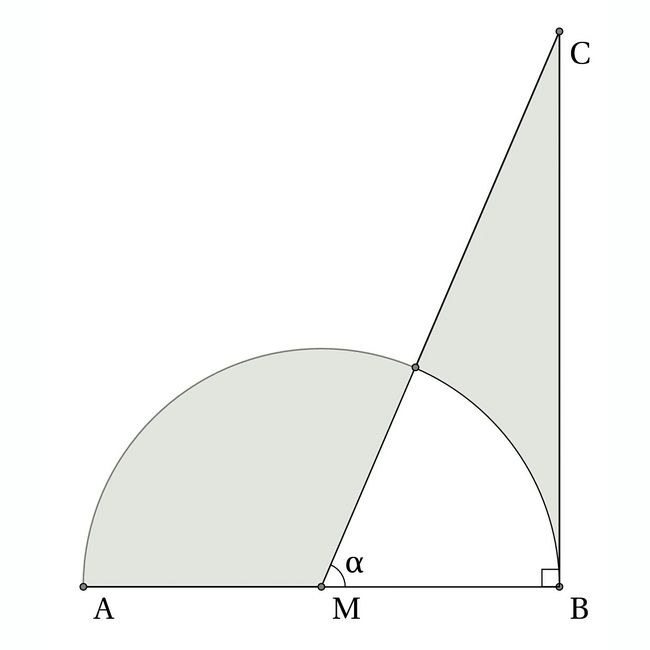# Equal area!

Geometry Level 2The above figure shows a semicircle with center $M$ and a right triangle $MBC$. The two shaded regions are equivalent. Find $\alpha$.

×